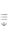AtomicDensityMatrix`
AtomicDensityMatrix`

# RotatingWaveTransformMatrix

RotatingWaveTransformMatrix[sys,{ω,tr}]

finds the transformation matrix suitable for applying the rotatingwave approximation on atomic system sys, assuming an optical field with angular frequency ω acting on transitions specified by tr.

RotatingWaveTransformMatrix[sys,{{ω1,tr1},{ω2,tr2},}]

finds the transformation matrix assuming optical fields with angular frequencies ωi acting on transitions specified by tri.

RotatingWaveTransformMatrix[sys,ω]

finds a heuristically generated transformation matrix appropriate for a single optical field.

# Details and Options

• The atomic system sys is specified as a list of AtomicState objects.
• Transitions are specified as described in the notes for RotatingWaveApproximation.
• The transformation matrix is constructed according to the method described in the notes for RotatingWaveApproximation.
• The unitary transformation obtained from RotatingWaveTransformMatrix can be supplied as the value of the TransformMatrix option for RotatingWaveApproximation. This is done automatically when is used. It can also be supplied as an argument to EffectiveHamiltonian.
• The following options can be given:
•  MethodAutomatic the method to use for performing the RWA TimeVariableAutomatic symbol used to represent the time variable

# Examples

open allclose all

## Basic Examples(2)

Define a two-level system:

Find a transformation matrix suitable for applying the rotating-wave approximation, assuming that a field of frequency ω couples the lower state 1 to the upper state 2:

For this system, RotatingWaveTransformMatrix can guess that state 1 is the lower state and state 2 is the upper state, so it is not necessary to specify the transition:

Here is an example with three levels and two fields.

Define a three level system:

Find a transformation matrix for the RWA assuming that Energy[a]<Energy[b]<Energy[c]:

If, instead, we assume that Energy[a]<Energy[c]<Energy[b], we obtain a different transformation matrix:

## Options(2)

### Method(1)

With , RotatingWaveTransformMatrix shifts the energy of the upper state to eliminate the optical frequency:

Method->"ShiftLowerState" can be used to instead shift the energy of the lower state:

### TimeVariable(1)

Find an RWA transformation matrix using a different time variable: